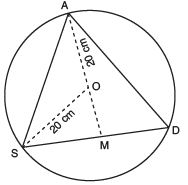Courses

# Value Based Questions- Circles Class 9 Notes | EduRev

## Mathematics (Maths) Class 9

Created by: Full Circle

## Class 9 : Value Based Questions- Circles Class 9 Notes | EduRev

The document Value Based Questions- Circles Class 9 Notes | EduRev is a part of the Class 9 Course Mathematics (Maths) Class 9.
All you need of Class 9 at this link: Class 9

Question 1. Amit, Deepak and Prabha have saved a good amount from their pocket money. They wish to donate it for a good cause. They sat on a round table of 20 cm radius, to decide the mode of donation. In the adjoining figure, Amit, Deepak and Prabha are sitting at A, S and D respectively, such that AS = SD = AD i.e., ∆ASD is an equilateral triangle.
(a) Find the distance between Amit, Deepak and Prabha.
(b) Which mathematical concept is used in the above problem?
(c) By donating the savings from the pocket money what values are depicted by Amit, Deepak and Prabha?Sol. (a) Since, AS = SD = AD [Given]
â ∆ASD is an equilateral triangle.
⇒ Perpendicular AM will pass through O.
â SM = (1/2) (SD) = x cm (say)                        [∵ Perpendicular from the centre to a chord, bisects the chord]
Now, in right angled ∆ASM, we have AM2 + SM=AS2
⇒ AM2 =AS2 – SM2
= (2 SM)2 – SM2                                    [∵ AS = SD and SM = (1/2) SD]
= 4SM2 – SM2 = 3 SM2 = 3x2
⇒ AM = √3x
Now, OM = AM – OA =                [ √3x – 20] cm
Also, in rt. ∆OBM, we have OS2 =SM2 + OM2
202 = x2 + OM2
⇒ 400 = x2 + ( √3x – 20)
⇒ 400 = x2 + 3x2 + 400 – 40 3x
⇒ 4x– 40 3x =0
⇒ 4x  (x – 10 √3= 0
⇒ x = 0 or x = 10√3
∵ x = 0 is not possible,
â x =10 3 cm
Now, SD = 2x cm = 2 × 10√3 cm = 20 √3 cm
⇒ Distance between Amit, Deepak and Prabha is 20√3 cm.
(b) Circles
(c)(i) Charity (ii) Habit of saving (iii) Taking right decision.

,

,

,

,

,

,

,

,

,

,

,

,

,

,

,

,

,

,

,

,

,

;